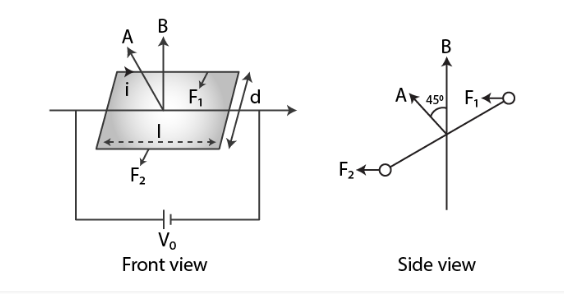# A rectangular conducting loop consists of two wires

Question:

A rectangular conducting loop consists of two wires on two opposite sides of length l joined together by rods of length d. The wires are each of the same

material but with cross-sections differing by a factor of 2. The thicker wire has a resistance R and the rods are of low resistance, which in turn are connected

to a constant voltage source Vo. The loop is placed in uniform a magnetic field B at 45oto its plane. Find τ, the torque exerted by the magnetic field on the loop

about an axis through the centres of rods.

Solution:Force and torque on the first wire is given as

F1 = i1l B sin 90o = V0/2R lB

τ1= d/2√2

F1 = V0ldB/2√2 R

τ = 1/4√2 V0AB/R